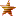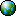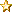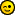December 12, 2019, 05:41:46 am
 News: Want to see a specific Tutorial? ASK!# Request for Calculator tutorial

 Pages:Author Topic: Request for Calculator tutorial  (Read 1028 times)
GarrisonRicketson
Hero MemberPosts: 583« on: March 11, 2011, 03:59:54 pm »

I was just looking at some old programs, and old posts,..and remembered,
I had been trying to make a calculator,...but never really did, ...I would like to see a tutorial on this, for qb64,..in the qb64 tutoriials, or here...
Where ever is most appropriate...
from Garry
 « Last Edit: March 11, 2011, 08:00:04 pm by GarrisonRicketson » Report SpamLogged

GarrisonRicketson
Hero MemberPosts: 583« Reply #1 on: March 11, 2011, 08:06:42 pm »

Ok, yea a simple one,..just add,multiply, divide  Twice now, the Calculator, that comes with windows, got deleted, as a virus, by my anti virus soft ware!,..but I don't need one that fancy...
Sorry I did not know that is one of your weak points, well I guess I do remember, you mentioned, math was a weak area, me too, that is why I need a calculator.
Thanks....
 Report SpamLogged

guest
Guest« Reply #2 on: March 11, 2011, 08:48:02 pm »

Hi all,
Here's the start of a Calculator Program with the theory first.
Quote
TO MAKE A CALCULATOR
Part I
Ok how do we make a calcuklator? Well we start with the basic functions that are

needed.
2] Subtract
3] Multiply
4] Divide
To make these functions we will need some data. We will obviously need the numbers
0123456789 and the symbols Add (+), Subtract (-), Multiply (*), Divide (\), Equals (=),clear
entry (c), clear all (a), Dot (.). So we will need to crate them as objects.
These will be objects that we click on with the mouse and by clicking on them we use
them. These objects need to be selected in the right sequence else we will create an error.
As humans if we selected the add symbol first and two numbers after we understand what it is
we wish to do. The computer can not do this. It needs defined and predictable actions to
respond to. So this means we have to have a set of rules that the program understands and
follows to arrive at the correct answer.
Before we come to the constructed actions of using these objects we need to define
the data types that will be used. An integer can be only minus 32,768 to 32,767. This size
may not be big enough for some operations. Also if a user enters a floating point figure (eg.
99.33) that will not work in an integer. We basically need two types LONG INTEGER(&) and
SINGLE(!).Somehow we need to detect if a user enters a DOT and make the appropriate type
selection based on that.
One way to allow any entry is to use strings\$. We would build the string of numbers
entered until the user selects a symbol. Before we react to the symbol we scan the string\$
for the dot. If we detect the dot then we have to use the FLOAT type. Next we would decode
the string into our first variable. So recapping the string gets converted into a FLOAT or a
SINGLE. The only other thing that need to be monitored is if the entered number is positive
or negative. This could potentially complicate it for us. The most obvious thing that i can
think of to differenciate between the symbol minus and negative is to use minus as an action
and then negative and positive as a condition. So in theory now we have to add 2 new symbols
to indicate positive and negative that only affects the sign of numbers.
Next is how the actions occur and get interpreted in the correct sequence. Normally a
user enters a number. This number is assumed to be positive unless he indicates its negative
with a different symbol selection. Next an operator is selected like addition. Next the user
enters a second number that may be positive or negative. Lastly the user selects a result
like equals.
Action1 + Action2 + Action3 + Action4 results in a calculation that appears in an output variable.

 « Last Edit: August 26, 2012, 05:42:51 pm by GarrisonRicketson » Report SpamLogged
SMcNeill
NewbiePosts: 3« Reply #3 on: August 26, 2012, 05:26:35 pm »

A very simple calculator program for you:
Code:
'Very Basic Calculator
'code by Steve McNeill
'NOTE:  Code is free for any use.  Modification, use, abuse, and obiliteration of code is perfectly acceptable.
'Use and modify as wanted, with no credits needed or expected.

DO
COLOR 0, 15
PRINT " "; '                                     This isn't important.  It's just here so we can line up our display better.
COLOR 15, 0
firstrun = 0
num1 = 0: operation\$ = "+"
DO
num2 = GetInput(operation2\$) '                Get our second number and next operation
SELECT CASE operation\$ '                      use our first number and its operation with the second number
CASE "+": total = num1 + num2 '           add
CASE "-": total = num1 - num2 '           subtract
CASE "*": total = num1 * num2 '           multiply
CASE "/": total = num1 / num2 '           divide
END SELECT
num1 = total: operation\$ = operation2\$ '      our first number is now the total, and our operation is what we wanted to do after the last number
IF firstrun = 0 THEN
firstrun = 1
PRINT " "; operation\$; " ";
ELSE
PRINT " = "; total '                          display our total
PRINT total; operation\$; " "; '               print our new first number, and the new operation we want with it.
END IF
LOOP UNTIL operation\$ = "=" '                     repeat the process until we finally hit enter or equal
PRINT total '                                     Print our final total
LOOP
END

FUNCTION GetInput (operation\$)
temp\$ = "": operation\$ = "": done = 0: period = 1
DO
a\$ = UCASE\$(INKEY\$)
SELECT CASE a\$
CASE CHR\$(8)
IF LEN(temp\$) > 0 THEN
IF RIGHT\$(temp\$, 1) = "." THEN period = 1
temp\$ = LEFT\$(temp\$, LEN(temp\$) - 1)
LOCATE , POS(0) - 1: PRINT " ";: LOCATE , POS(0) - 1
END IF
CASE "1", "2", "3", "4", "5", "6", "7", "8", "9", "0": temp\$ = temp\$ + a\$: PRINT a\$;
CASE CHR\$(13), "=": done = 1: IF temp\$ = "" THEN operation\$ = "" ELSE operation\$ = "="
CASE ".": IF period THEN period = 0: temp\$ = temp\$ + ".": PRINT ".";
CASE "-": IF temp\$ = "" THEN temp\$ = "-": PRINT "-"; ELSE done = 1: operation\$ = "-"
CASE "+": IF temp\$ = "" THEN operation\$ = "" ELSE done = 1: operation\$ = "+"
CASE "*", "X": IF temp\$ = "" THEN operation\$ = "" ELSE done = 1: operation\$ = "*"
CASE "/": IF temp\$ = "" THEN operation\$ = "" ELSE done = 1: operation\$ = "/"
END SELECT
LOOP UNTIL done
IF LEN(temp\$) = 0 AND operation\$ = "" THEN ' we're finished for good
END
ELSE
GetInput = VAL(temp\$)
END IF
END SUB

Feel free to change, modify, expand, and use as needed.  I tried to keep it simple so no one would have a problem understanding how it works, so all it does at the moment is add, subtract, multiply, and divide -- in sequential order.   12 + 1 * 3 = 39

Think of it along the lines of one of those 99 cent calculators you'd buy from the Dollar General store.Report SpamLogged
GarrisonRicketson
Hero MemberPosts: 583« Reply #4 on: August 26, 2012, 05:56:26 pm »

Thanks for posting this, it worked good. I compiled it with qb64 for Linux,
from Garry
 Report SpamLogged

 Pages: